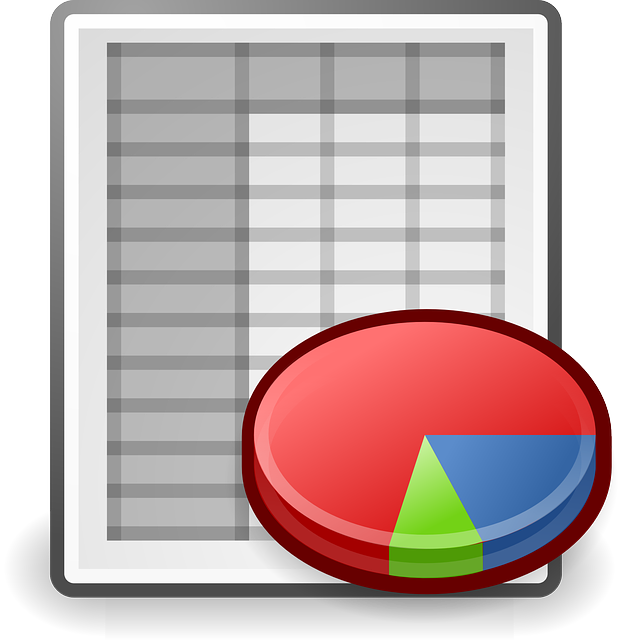# Determining the compass deviation using a faraway object

November 4, 2016 SorinMStamate

### Determining the compass deviation using a faraway object:

To determine compass deviation, we can use a single object, enough faraway, where we observe the compass bearings in the 8 (eight) courses.

Magnetic bearings to the object can be determined, depending on the situation, by various methods.

After that we calculate the compass deviation and the compass deviation table.

The maximum error in determining the deviation will be the error in the bearing to the object.

There are various mathematical relationships between the distance to the object, the radius of gyration and error in determining the deviation.

The file contains various examples that can be deleted and edited with your data.Determining the compass deviation using a faraway object _ v1.0